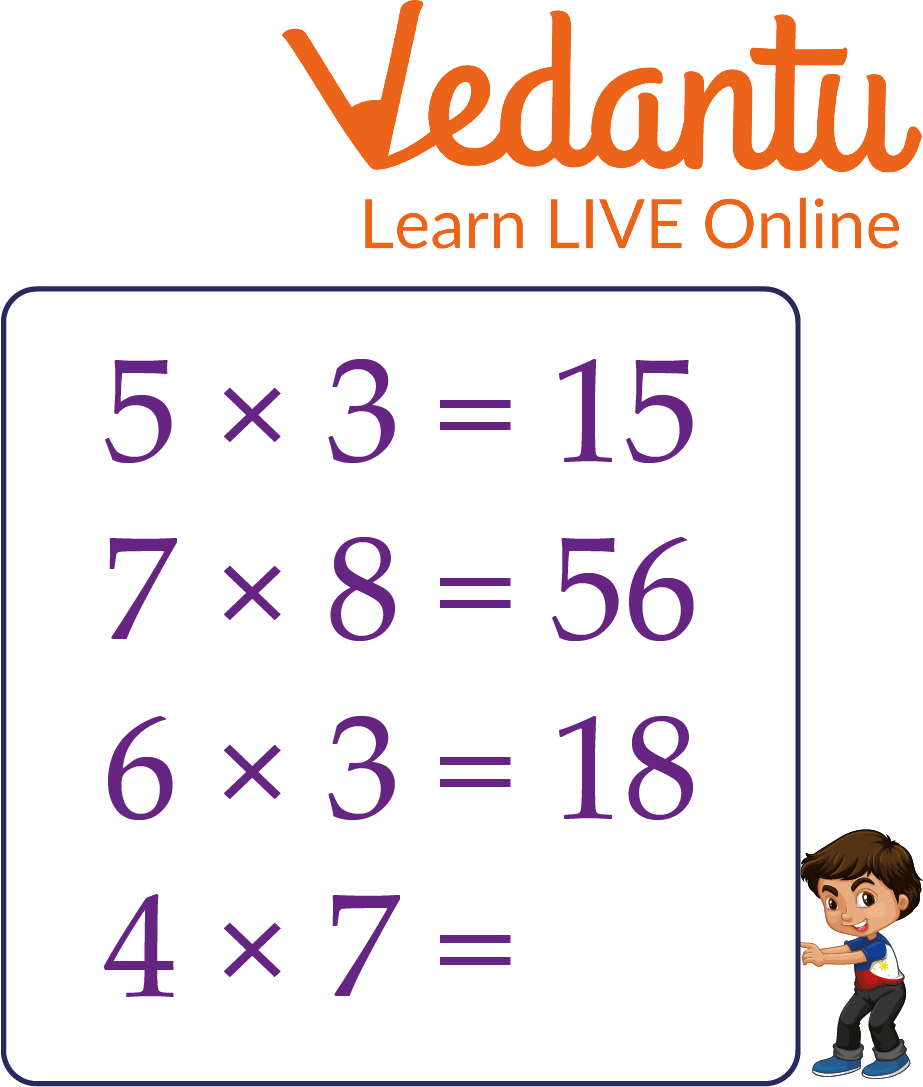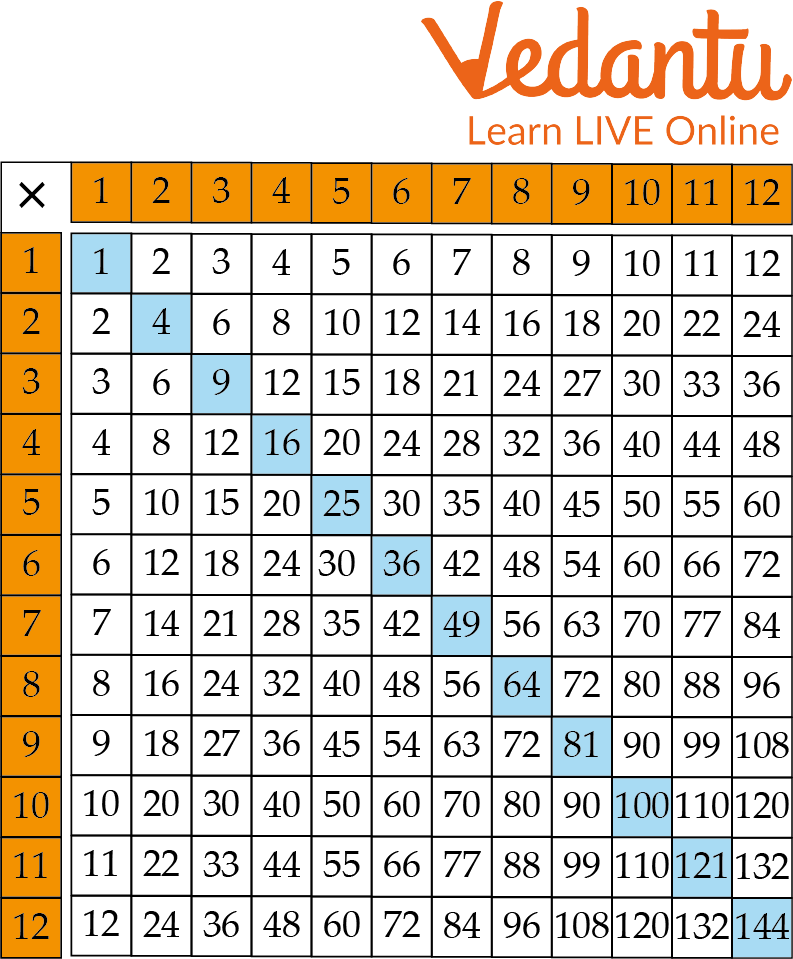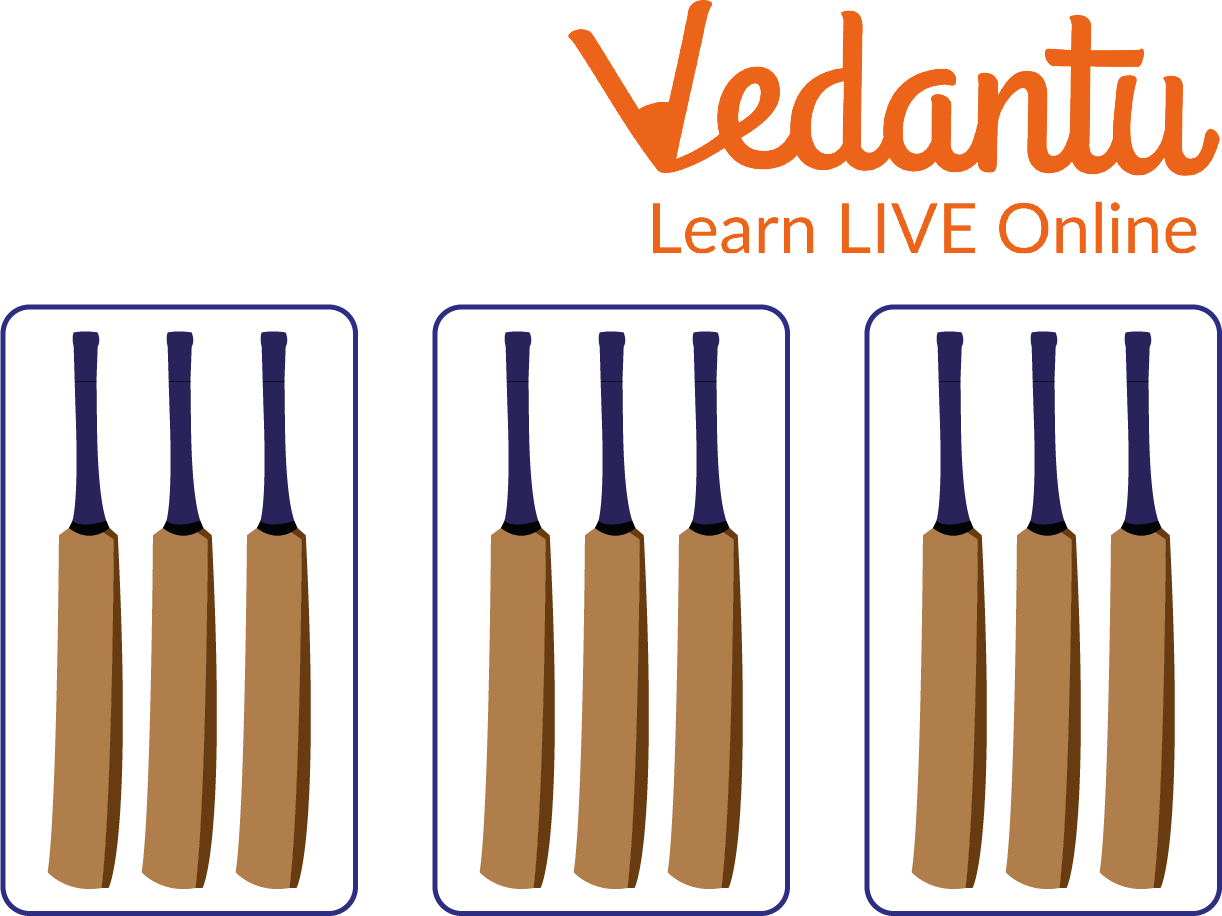Courses
Courses for Kids
Free study material
Free LIVE classes
More

# Multiplication Facts## Introduction to Multiplication

As we all know what multiplication is and how we solve questions related to multiple class 2. But did you know that we also call the solution of any multiplication equation a fact? Some standard multiplication facts are needed to follow for multiplying numbers and there are several methods by which we can find out the multiplication facts. The repeated addition of one particular number is expressed by multiplication. Some maths sheets for class 2 are given below for practice.

## Multiplication Fact

A multiplication fact is considered the answer to a multiplication calculation when a number is multiplied by a different set of numbers. It follows some patterns to multiply two numbers and write their products aside.

Here are some examples of multiplication facts:

• 2 × 3 = 6 (the multiplication fact is 6)

• 7 × 5 = 35 (the multiplication fact is 35)

• 10 × 2 = 20 (the multiplication fact is 20).Multiplication Fact

## How to Write Multiplication Facts for Class 2?

You can write multiplication facts by writing out multiplication calculations.

For example:

• 12 x 10 = 120

• 3 x 7 = 21

• 8 x 6 = 48

Multiplication facts can also be written by different methods of multiplication.

For example, repeated addition is a way of finding multiplication facts. One can repeatedly add a number to reach the answer. 2 × 4 is the same as 2 + 2 + 2 + 2 = 8. The multiplication fact, in this case, is 8.

## How to Learn Multiplication Facts for Class 2?

One of the best ways to learn multiplication facts is through repetition since it helps children to form a good and strong memory of multiplication facts.Learning Method

Given are some ways to learn multiplication facts and their multiplication tables:

1. Using a multiplication square that shows the multiplication facts for numbers in the times table. This provides a great visual aid for learning times tables and multiplication facts.

2. Reciting the multiplication table forwards and backwards. Reciting it out loud helps to reinforce one's memory of basic multiplication facts.

3. Practising skip counting. Skip counting is a type of multiplication by repeated addition. For eg, skip counting by 2 is the same as the table of 2: 2, 4, 6, 8, 10, etc.

## Solved Examples of Multiples Class 2

Q 1. If a bowl contains 25 marbles, each group should have 5 marbles. Then how many such groups can be formed?

Ans. The total number of marbles in a bowl is = 25

5 marbles are used to make a group, i.e. the number of groups is calculated using the formula:

Number of groups = Total number of marbles in a bowl divided by the number of marbles required to make a group

= $\dfrac{25}{5}$

= 5

One can also do it by the process of repeated addition, i.e. add 5 to itself 5 times, it will result in 25, i.e. 5+5+5+5+5 = 25.

Thus, the required number of groups of marbles are 5.

Q 2. How many groups of bats are there?Solved Example

Ans. The total number of bats in 3 groups is calculated by adding 3 to itself three times, i.e. 3 + 3 + 3 = 9. This can also be found by multiplying, three by three, i.e. 3 × 3.

Thus, the required number of bats in the group is 9.

## Practice Problems

Q1. How many brinjals are there, if the number of groups of brinjals is 144 and there are 8 brinjals in each one?

Q2. How many groups of two apples each can be made using 166 apples?

Q3. How many groups can be made of 240 carrots, if there should be 12 carrots in a group?

## Class 2 Maths Multiplication Worksheets

Below given is the worksheet maths for class 2, which helps the students to evaluate their learning of the multiplication facts.

(a) $7 \times 11$ (Ans: 77)

(b) $7 \times 2$ (Ans: 14)

(c) $8 \times 9$ (Ans: 72)

(d) $9 \times 5$ (Ans: 45)

(e) $7 \times 10$ (Ans: 70)

(f) $6 \times 12$ (Ans: 72)

(g) $10 \times 30$ (Ans: 300)

(h) $4 \times 11$ (Ans: 44)

(i) $2 \times 25$ (Ans: 50)

(j) $3 \times 15$ (Ans: 45)

## Summary

In this article, we learned about multiplication facts and some basic facts that are needed for multiplying two numbers such as the repeated addition method where we make groups of the given number and then add them to get the fact. We also learned about how to write and learn multiplication facts. Some unsolved maths sheets for class 2 are also given. Worksheet maths for class 2 serves as a useful tool in evaluating the progress of students.

Last updated date: 01st Oct 2023
Total views: 92.1k
Views today: 0.92k

## FAQs on Multiplication Facts

1. How many multiplication facts are there?

There are infinite numbers of multiplication facts because numbers are infinite. Since, there are infinite numbers. So based on that infinite multiplication can be formed and their facts too. The table is used to represent multiplication facts and is often called the multiplication or times table.

2. Who brought the concept of multiplication?

The concept of multiplication was brought up by the famous mathematician, The Babylonians. Nearly, four thousand years ago, the Babylonians gave the concept of multiplication.

3. What are the other symbols for multiplication?

The other symbols are: (⋅), (×), or (∗) between two mathematical expressions, denoting multiplication of the second expression by the first.